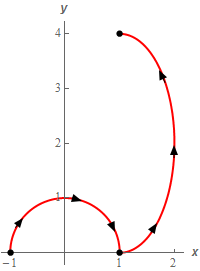Paul's Online Notes
Home / Calculus III / Line Integrals / Fundamental Theorem for Line Integrals
Show Mobile Notice Show All Notes Hide All Notes
Mobile Notice
You appear to be on a device with a "narrow" screen width (i.e. you are probably on a mobile phone). Due to the nature of the mathematics on this site it is best views in landscape mode. If your device is not in landscape mode many of the equations will run off the side of your device (should be able to scroll to see them) and some of the menu items will be cut off due to the narrow screen width.
Assignment Problems Notice
Please do not email me to get solutions and/or answers to these problems. I will not give them out under any circumstances nor will I respond to any requests to do so. The intent of these problems is for instructors to use them for assignments and having solutions/answers easily available defeats that purpose.

If you are looking for some problems with solutions you can find some by clicking on the "Practice Problems" link above.

### Section 16.5 : Fundamental Theorem for Line Integrals

1. Evaluate $$\displaystyle \int\limits_{C}{{\nabla f\centerdot d\vec r}}$$ where $$f\left( {x,y} \right) = 5x - {y^2} + 10xy + 9$$ and $$C$$ is given by $$\displaystyle \vec r\left( t \right) = \left\langle {\frac{{2t}}{{{t^2} + 1}},1 - 8t} \right\rangle$$ with $$- 2 \le t \le 0$$.
2. Evaluate $$\displaystyle \int\limits_{C}{{\nabla f\centerdot d\vec r}}$$ where $$\displaystyle f\left( {x,y,z} \right) = \frac{{3x - 8y}}{{z - 6}}$$ and $$C$$ is given by $$\vec r\left( t \right) = 6t\,\vec i + 4\vec j + \left( {9 - {t^3}} \right)\vec k$$ with $$- 1 \le t \le 3$$.
3. Evaluate $$\displaystyle \int\limits_{C}{{\nabla f\centerdot d\vec r}}$$ where $$f\left( {x,y} \right) = 20y\cos \left( {x + 3} \right) - y{x^3}$$ and $$C$$ is right half of the ellipse given by $$\displaystyle {\left( {x + 3} \right)^2} + \frac{{{{\left( {y - 1} \right)}^2}}}{{16}} = 1$$ with clockwise rotation.
4. Compute $$\displaystyle \int\limits_{C}{{\vec F\centerdot d\vec r}}$$ where $$\vec F = 2x\,\vec i + 4y\,\vec j$$ and $$C$$ is the circle centered at the origin of radius 5 with the counter clockwise rotation. Is $$\displaystyle \int\limits_{C}{{\vec F\centerdot d\vec r}}$$ independent of path? If it is not possible to determine if $$\displaystyle \int\limits_{C}{{\vec F\centerdot d\vec r}}$$ is independent of path clearly explain why not.
5. Compute $$\displaystyle \int\limits_{C}{{\vec F\centerdot d\vec r}}$$ where $$\vec F = y\,\vec i + {x^2}\,\vec j$$ and $$C$$ is the circle centered at the origin of radius 5 with the counter clockwise rotation. Is $$\displaystyle \int\limits_{C}{{\vec F\centerdot d\vec r}}$$ independent of path? If it is not possible to determine if $$\displaystyle \int\limits_{C}{{\vec F\centerdot d\vec r}}$$ is independent of path clearly explain why not.
6. Evaluate $$\displaystyle \int\limits_{C}{{\nabla f\centerdot d\vec r}}$$ where $$f\left( {x,y,z} \right) = z{x^2} + x{\left( {y - 2} \right)^2}$$ and $$C$$ is the line segment from $$\left( {1,2,0} \right)$$ to $$\left( { - 3,10,9} \right)$$ followed by the line segment from $$\left( { - 3,10,9} \right)$$ to $$\left( {6,0,2} \right)$$.
7. Evaluate $$\displaystyle \int\limits_{C}{{\nabla f\centerdot d\vec r}}$$ where $$f\left( {x,y} \right) = 4x + 3x{y^2} - \ln \left( {{x^2} + {y^2}} \right)$$ and $$C$$ is the upper half of $${x^2} + {y^2} = 1$$ with clockwise rotation followed by the right half of $$\displaystyle {\left( {x - 1} \right)^2} + \frac{{{{\left( {y - 2} \right)}^2}}}{4} = 1$$ with counter clockwise rotation. See the sketch below.